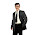## Saturday, 9 June 2012

### CBSE - Class 9 - Maths - CH1 - Number Systems (Ex 1.5)Still it is unknown, if ( π + e) is rational or not?

Important Points about Rational and Irrational Numbers

1. The sum or difference of a rational number and an irrational number is always an irrational number.
If a is a rational number and √b is an irrational number then,

(i) a + √b is irrational
(ii) a - √b is irrational
(iii) a√b is irrational
(iv) a ÷ √b is irrational
(v) √b ÷ a is irrational

2. The product or quotient of non-zero rational number and an irrational number is also an irrational number.

3. Sum, difference, product or quotient of two irrational numbers need not be irrational. The result may be rational or irrational.
e.g. √m and √n are irrational. √m x √n = √(mxn) may be rational or irrational.

4. If a is positive rational number then surd  n√a is irrational.

5.

Exercise 1.5

Q1: Classify the following numbers as rational or irrational:
(i) 2 − √5
(ii) (3 + √(23)) − √(23)
(iii) 2√7/7√7
(iv) 1/√2
(v) 2π

Answer:

(V) 2π = 2 x 3.141528... = 6.283056...
Since it is non-terminating, non-recurring, it is an irrational number.

Q2: Simplify each of the following expressions:

(i) (3 + √3) (2 + √2)
(ii) (3 + √3) (3 − √3)
(iii) (√5 + √2)2
(iv) (√5 - √2)(√5 + √2)

Answer:
(See the formula listed above in point 5)

(i) (3 + √3) (2 + √2) = 3x2 + 3√2 + 2√3 + √3x√2 = 6 + 3√2 + 2√3 + √6

(ii) (3 + √3) (3 − √3) = (3)2 - (√3)2= 9 - 3 = 6
(iii) (√5 + √2)2 = (√5)2 + (√2)2 + 2(√5)(√2) = 5 + 2 + 2√10 = 7 + 2√10

(iv) (√5 - √2)(√5 + √2) = (√5)2 - (√2)2= 5 - 2 = 3

Q3. Recall, π is defined as the ratio of the circumference (say c) of a circle to its diameter (say d). That is, p = c/d. This seems to contradict the fact that p is irrational. How will you resolve this contradiction?

Answer: It does not contradict. When we measure some value with a scale or device, we often approximate the values. i.e. π is almost equal to 22/7 (i.e. π ≈ 22/7).

In fact π = 3.141528… while 22/7 = 3.142857…

Q4. Represent √(9.3) on the number line.

Answer:

1. Draw a line AB = 9.3 units on number line.
2. Extend B to C such that BC = 1 unit.
3. Find the mid-point AC say point D and draw a circle on OC.
4. Draw a perpendicular to line AC passing through point B. Let it intersect the circle at F.
5. Taking B as centre and BF as radius, draw an arc intersecting number line at G. BF is √(9.3)

Q5: Rationalise the denominators of the following

Q6: Solve √8 x √18

Answer:  √8 x √18 = √(8 x 18) = √(144) = √(12)2  = 12

.#### 8 comments:

1.interesting..will it be available for all chapters. will be of great use to me to teach my son who is in the 9th standard.

1.Thanks for appreciation. We are a small team. We do our best to provide as many topics as possible.

2.we all are waiting for you to publih all the other chapters tooo.recurit some more members and u r truly awesome

2.i am understanding every thing thank you for the presentation

3.i can easily understand you presentation

4.nice i like it cant we copy it

5.nice i like it cant we copy it

1.we can just right click the image part and save it or copy

We love to hear your thoughts about this post!